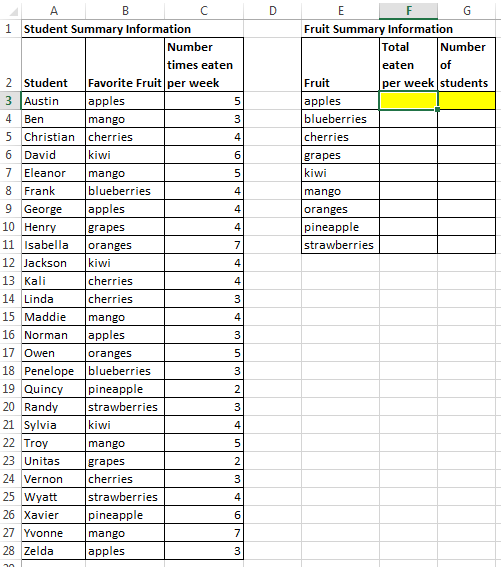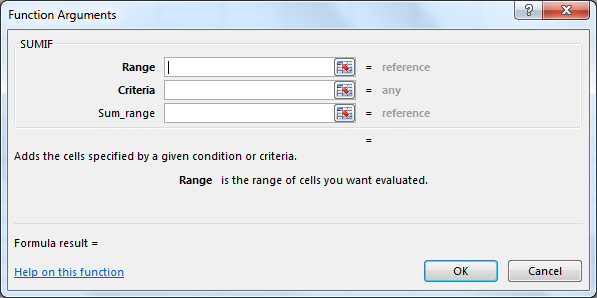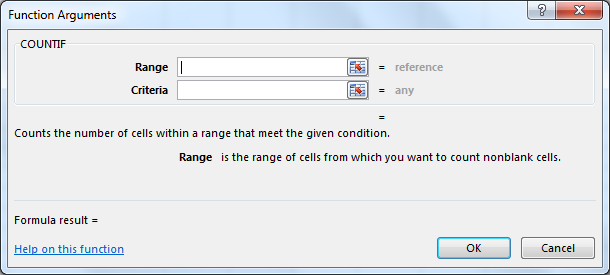# Quantitative Literacy Support Center

## The following presents an Excel tutorial for working with complex functions.

Two complex functions that can come in very handy are SUMIF and COUNTIF. These functions allow you to perform a calculation if certain criteria are met. The key to working with complex functions is to use the ‘Insert Function’ dialog box effectively.

In the following example, we are provided student summary information: a list of students, their favorite fruits, and how often they eat the fruit each week (see Complex_Functions_Starting_Wkbk.xlsx). We would like to obtain summary information for the fruit.

(1)   How much of a given fruit is eaten each week?

(2)   How many students choose the given fruit as their favorite?For (1), we need to use the SUMIF function in cell F3. We want to calculate the sum of the number of times eaten per week (column C) for each fruit given in column B. Place your cursor in cell F3, then click the ‘Insert Function’ button. Type SUMIF, click GO, then double-click the SUMIF function in the results list. You will see the following dialog box:First, we need to select the Range. This is the range of cells that you want to evaluate for a certain criteria. For this example, we need to select the range that contains the names of the fruit, B3:B28. Next, we need to set the Criteria. The criteria is the condition that needs to be met in order to calculate the sum. For this example, we need to specify the single cell that indicates the specific fruit that we are looking for to use for the calculation. This fruit is indicated in our ‘Fruit Summary Information’ table, column E. In the criteria box, type E3. Finally, we need the Sum_range. This box should contain the range of cells that we want to sum. For this example, we want to sum the number of times eaten per week. We provide the full range for this information, C3:C28. The SUMIF function will then only sum the cells within the C3:C28 range if the criteria of the specific fruit is met. In other words, in cell F3, the SUMIF function only sums the number of times eaten per week if the word “apples” is given in the range B3:B28.

For (2), we need to use the COUNTIF function in cell G3. We want to count how many students have chosen a fruit as their favorite for each fruit given in column B. Place your cursor in cell G3, then click the ‘Insert Function’ button. Type COUNTIF, click GO, then double-click the COUNTIF function in the results list. You will see the following dialog box:First, we need to select the Range. This is the range of cells that you want to evaluate for a certain criteria. For this example, we need to again select the range that contains the names of the fruit, B3:B28. Next, we need to set the Criteria. The criteria is the condition that needs to be met in order to be included in the count. For this example, we need to specify the single cell that indicates the specific fruit that we are looking to count how many times the fruit is indicated as the favorite. This fruit is indicated in our ‘Fruit Summary Information’ table, column E. In the criteria box, type E3. The COUNTIF function will then only count how many cells within the C3:C28 range indicate the criteria of the specific fruit. In other words, in cell G3, the COUNTIF function only counts the number of times the word “apples” is given in the range B3:B28.

The solution for this tutorial is provided in Complex_Functions_Completed_Wkbk.xlsx.